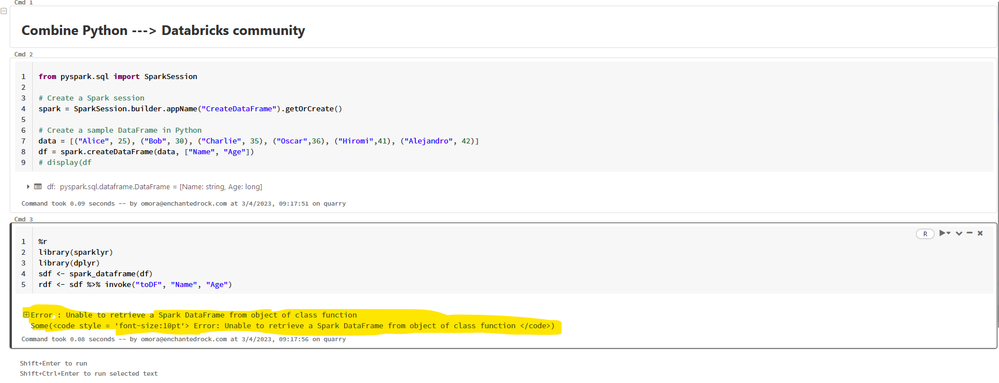cancel
Showing results for
Did you mean:

## Combine Python + R in data manipulation in Databricks NotebookNew Contributor II

Want to combine Py + R

from pyspark.sql import SparkSession

spark = SparkSession.builder.appName("CreateDataFrame").getOrCreate()

# Create a sample DataFrame

data = [("Alice", 25), ("Bob", 30), ("Charlie", 35), ("Oscar",36), ("Hiromi",41), ("Alejandro", 42)]

df = spark.createDataFrame(data, ["Name", "Age"])

display(df)

And R

%r

install.packages("sparklyr", version ="1.8.0")

library(sparklyr)

# Connect to the same Spark cluster

sc <- spark_connect(master = "yarn-client", version = "1.8.0"

)

But I have the error

**Error in spark_connect_gateway(gatewayAddress, gatewayPort, sessionId, : Gateway in localhost:8880 did not respond.

Try running

options(sparklyr.log.console = TRUE)

followed by

sc <- spark_connect(...)

for more debugging info. Some( Error in spark_connect_gateway(gatewayAddress, gatewayPort, sessionId, : Gateway in localhost:8880 did not respond. )**

Any Idea how can I combine both programming Languages in Databricks notebook?

2 REPLIES 2Anonymous
Not applicable

@Oscar CENTENO MORA​ :

To combine Py and R in a Databricks notebook, you can use the magics command %python and %r

to switch between Python and R cells. Here's an example of how to create a Spark DataFrame in Python and then use it in R:

``````from pyspark.sql import SparkSession

# Create a Spark session
spark = SparkSession.builder.appName("CreateDataFrame").getOrCreate()

# Create a sample DataFrame in Python
data = [("Alice", 25), ("Bob", 30), ("Charlie", 35), ("Oscar",36), ("Hiromi",41), ("Alejandro", 42)]
df = spark.createDataFrame(data, ["Name", "Age"])

# Use the %python magic to switch to a Python cell
%python

# Convert the Python DataFrame to an R DataFrame using sparklyr
library(sparklyr)
library(dplyr)
sdf <- spark_dataframe(df)
rdf <- sdf %>% invoke("toDF", "Name", "Age")

# Use the %r magic to switch to an R cell
%r

# Print the R DataFrame
print(rdf)``````

Note that the sparklyr package must be installed in the R environment using the install.packages()

function, as shown in your example. Also, make sure that the Spark cluster is running and accessible from your notebook.New Contributor II

Hello,

I did exactly that, and no, the %r or %python, which indicate in each command what the programming language is, still gives an error. This is the error:What you mentioned was in the guides and forums, but testing it still doesn't give a correct result.Welcome to Databricks Community: Lets learn, network and celebrate together

Join our fast-growing data practitioner and expert community of 80K+ members, ready to discover, help and collaborate together while making meaningful connections.